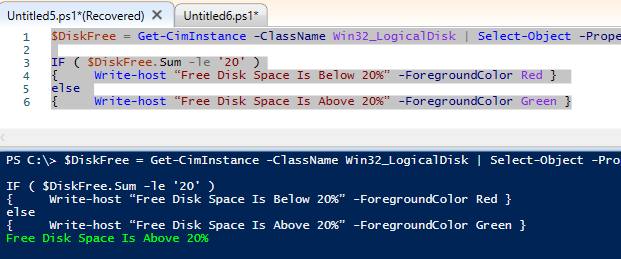# How To Use The IF Statement In Powershell: 13 Examples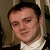Jason Barrett | | Misc

I write powershell scripts a lot for my work so I decided to create this page to teach others how to use the IF command.

In this guide I will start of with very easy IF commands and will then progress with more advanced commands.

With all the commands below you can copy and paste them in to your powershell.

## Powershell IF Examples

Listed below are all of the IF commands I have used within powershell

### 1. If Condition Is True

This Will Do = If Condition is True = Do Something

In the example below, the \$FileCheck variable is created with a test-path command, if the file is found the \$FileCheck variable will be set to true.

Then the if ( \$FileCheck ) is checking of the value is true, if it is true then it will output File Was Found

\$FileCheck = test-pathPathC:\Temp\Testfile.txt

if ( \$FileCheck )

{ Write-HostFile Was Found”  –ForegroundColor Green }

In the below example you can see that the file was found and the text File Was Found was outputted.  I like to change the colour of the text that was output with Green for good / found or red error / not found.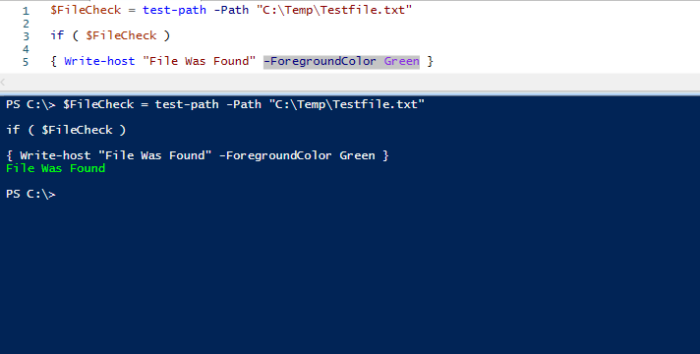### 2. If Condition Is True Else

This Will Do = If Condition is True = Do Something else if not true Do something else.

By adding the else command we can do something else if the condition is not True.

Below I have added 2 more lines to the original script.  Now if the \$FileCheck variable is false it will run the line after the else command.

\$FileCheck = test-path -Path “C:\Temp\Testfile.txt”

if ( \$FileCheck )

{ Write-host “File Was Found” -ForegroundColor Green }
else

In the screenshot below I have deleted the file C:\Temp\Testfile.txt which will make the \$FileCheck variable to be false.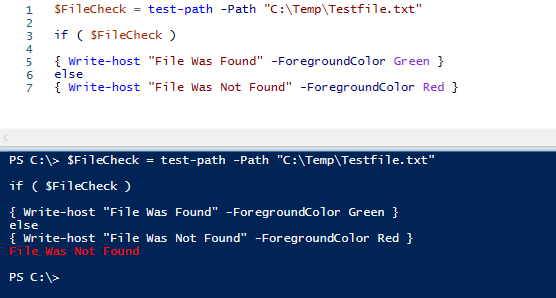### 3. If Condition Is False

This Will Do = If Condition is False = Do Something

If you want to check if a condition is false you can use the -eq \$false code to check this.

In the below example if the file C:\Temp\Testfile.txt is missing it will output the text File Was Not Found

\$FileCheck = test-path -Path “C:\Temp\Testfile.txt”

if ( \$FileCheck -eq \$false )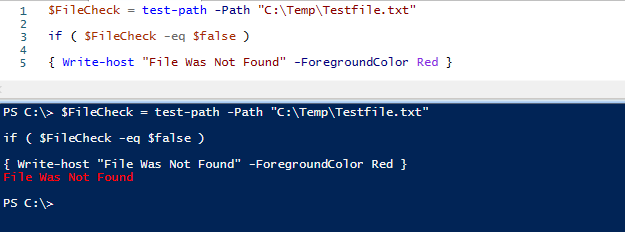### 4. If Condition Equals Value

This Will Do = If Condition Equals Value = Do Something

If you want to check if a condition equals a custom value then you can use the below code.

In the below example the if statement checks if \$FavouriteColour equals Purple, if it does it outputs Favourite Colour Is Purple else it outputs Favourite Colour Is Not Purple

\$FavouriteColour = “Purple”

If ( \$FavouriteColour -eq “Purple” )

{ Write-host “Favourite Colour Is Purple” -ForegroundColor Yellow }
else
{ Write-host “Favourite Colour Is Not Purple” -ForegroundColor Yellow }

Below we can see that the condition did equal Purple.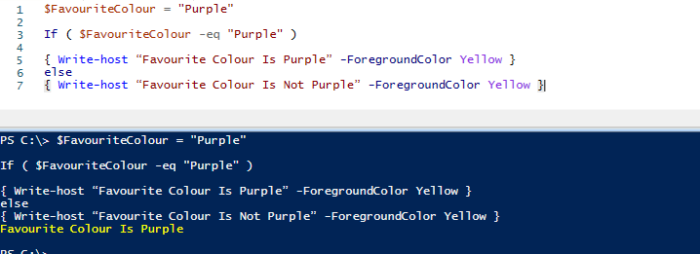### 5. If Condition Like Value

This Will Do = If Condition like value = Do Something

In some cases you might want to match only some of the variable.  In the below example I want to find anything with the text error.

\$Result = “Error 404”

If ( \$Result -like “Error%” )

{ Write-host “No Error Found” -ForegroundColor Green }
else
{ Write-host “Error Found”ForegroundColor Red }

The below condition matched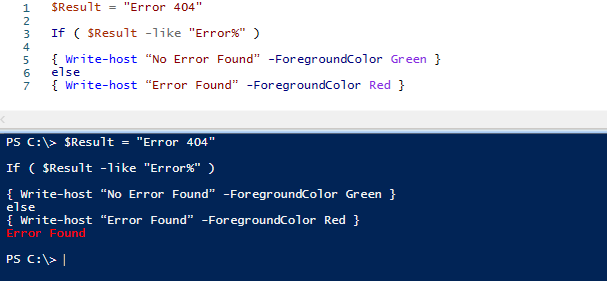### 6. If Condition Not Like Value

This Will Do = If Condition not like value = Do Something

In the below example if the \$Result variable does not contain Success it will show the text Success Not Found

\$Result = “http 404”

If ( \$Result -notlike “Success%” )

else
{ Write-host “Success Found” -ForegroundColor Green }

In the example below Success was not found in \$Result so it showed the text Error Found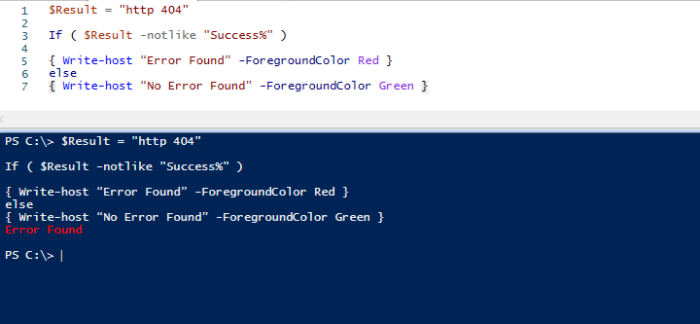### 7. If Condition Not Equal

This Will Do = If Condition is not equal to = Do Something

Using the -ne (Not Equal) operator we can then catch a variable that is not equal to.

Below the \$Score variable is set to 100, if the score is not 100 the text “Score Is Not Equal To 100” will show

\$Score = 100

If ( \$Score -ne 100 )

{ Write-host “Score Is Not Equal To 100” -ForegroundColor Yellow }
else
{ Write-host “Score Is Equal To 100” -ForegroundColor Yellow }

The \$Score variable is set to 100 so the script outputs “Score Is Equal To 100”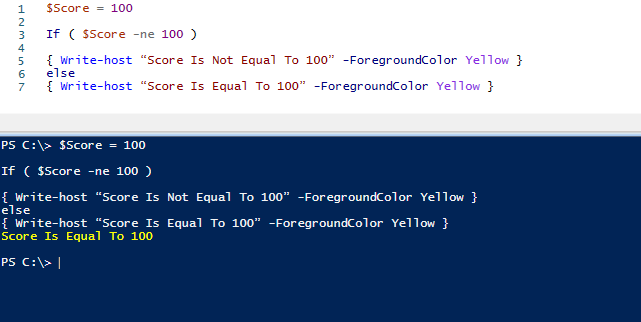### 8. If Condition Greater Than

This Will Do = If Condition is greater than = Do Something

Using the -gt (Greater Than) operator we can create an IF statement to check numbers if they are greater than a value.

The below script will check if the \$Score is above 100 and output accordantly.

\$Score = ‘1

If ( \$Score -gt ‘100)

{ Write-host “Score Is More Than 100” -ForegroundColor Yellow }
else
{ Write-host “Score Is Less Than 100” -ForegroundColor Yellow }

Below the score is less than 100 so the text “Score Is Less Than 100” shows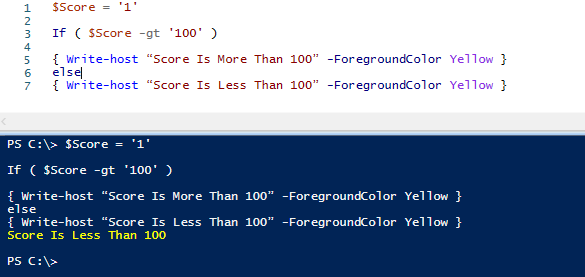### 9. If Condition Greater Than Or Equal

This Will Do = If Condition is equal or greater than = Do Something

Similar to the previous If statement but we replace -gt with -ge.

\$Score = ‘100

If ( \$Score -ge ‘100)

{ Write-host “Score Is 100 or More” -ForegroundColor Yellow }
else
{ Write-host “Score Is Less Than 100” -ForegroundColor Yellow }

### 10. If Condition Less Than

This Will Do = If Condition is less then or equal to = Do Something

By using the -le (Less Than Or Equal To)

\$Score = ‘99

If ( \$Score -le ‘100‘ )

{ Write-host “Score Is 100 or More”ForegroundColor Yellow }
else
{ Write-host “Score Is Less Than 100”ForegroundColor Yellow }

### 11. IF One of Multiple Variables

This Will Do = If one condition is met out of multiple = Do Something

If you have more than one variable you can use the below code to detect if one variable matches.

\$Sex = ‘Male’
\$Age = ’29’

If ( \$Sex -eq ‘Male’ -or \$Age -ge ’30’ )

{ Write-host “Result is Either Male, Over 30 or both” -ForegroundColor Green }
else
{ Write-host “No Match” -ForegroundColor Red }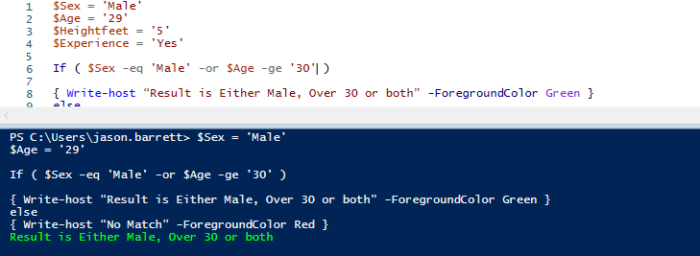With this example there is a match because the sex is male. Only one match is required because we put the -or operator.  You can put as many -or operators as you with, such as

\$Sex = ‘Male
\$Age = ‘29
\$Heightfeet = ‘5
\$Experience = ‘Yes

If ( \$Sex -eq ‘Male’ -or \$Age -ge ’30’ -or \$Heightfeet -ge ‘6’ -or \$Experience -eq ‘Yes’ )

### 12. IF Multiple Variables Met

This Will Do = If multiple conditions are met out of multiple = Do Something

By adding -and will require that all conditions are met.  The below condition is not met because the \$Age is 29 but the condition is 30+.

\$Sex = ‘Male’
\$Age = ’29’

If ( \$Sex -eq ‘Male’ -and \$Age -ge ’30’ )

{ Write-host “Result Male + 30 or over” -ForegroundColor Green }
else
{ Write-hostNo Match-ForegroundColor Red }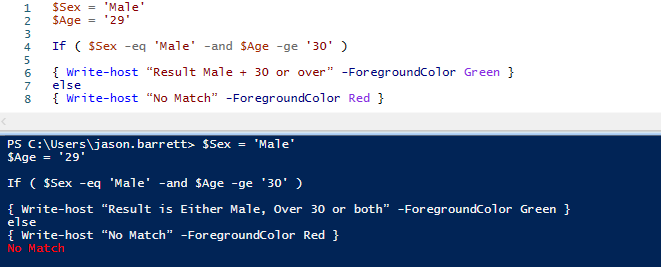### 13. If Sting Is Blank or Null

This Will Do = If the variable being used for the IF command is blank or null = Do Something

\$Result =

IF ([string]::IsNullOrWhitespace(\$Result))
{ Write-host “String Is Blank”ForegroundColor Yellow }
else
{ Write-host “String Is Not Blank” -ForegroundColor Yellow }

Above the \$Result variable is blank which enabled the IF command to output String Is Blank

## More Advanced Powershell If Commands

The above powershell commands are the basic ones that we will generally use.  Below I have pasted some of the more advanced IF commands that I have used with my work.

### 1. If Windows Service Is Running Status

This Will Do = Check if a windows service is running = if stopped the script will try to restart the service.

First thing we need to do is get the name of the windows service you want to use.  You can do this by typing “Get-service -name” then the list of services will be listed, in the below example I will be using the windows time service (W32Time).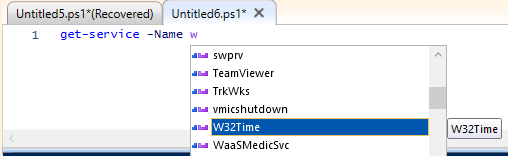Below is the powershell code to check if the W32Time service is stopped.  If the service is stopped the text “Service is Stopped, Starting” will appear and the service will also be started using the start-service command.

\$ServiceStatus = get-service -Name W32Time

IF ( \$ServiceStatus.Status -eq ‘Stopped’ )

{ Write-host “Service is Stopped, Starting” -ForegroundColor Red
Start-Service -Name W32Time }
else
{ Write-host “Service Is Started” -ForegroundColor Green }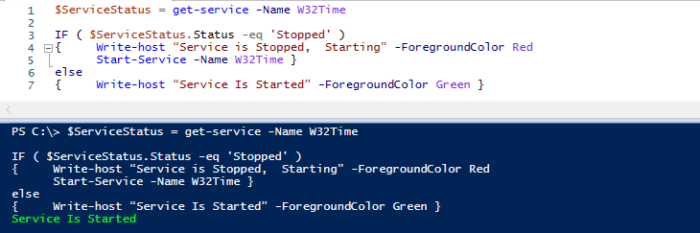### 2. If Free Disk Space Is Below 20%

This Will Do = Will check if the free disk space on your system is below a set value (Below 20%)

\$DiskFree = Get-CimInstance -ClassName Win32_LogicalDisk | Select-Object -Property DeviceID,@{‘Name‘ = ‘FreeSpace (GB)‘; Expression= { [int](\$_.FreeSpace / 1GB) }} | Measure-Object -Property ‘FreeSpace (GB)’ -Sum

IF ( \$DiskFree.Sum -le ‘20‘ )
{ Write-host “Free Disk Space Is Below 20%” -ForegroundColor Red }
else
{ Write-host “Free Disk Space Is Above 20%” -ForegroundColor Green }

If you want to change the 20% check to another value simply change the 20 value in IF ( \$DiskFree.Sum -le ‘20‘ ) to what you need.